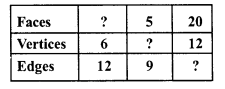# RD Sharma Class 8 Solutions Chapter 19 Visualising Shapes Ex 19.1

In this chapter, we provide RD Sharma Class 8 Solutions Chapter 19 Visualising Shapes Ex 19.1 for English medium students, Which will very helpful for every student in their exams. Students can download the latest RD Sharma Class 8 Solutions Chapter 19 Visualising Shapes Ex 19.1 Maths pdf, free RD Sharma Class 8 Solutions Chapter 19 Visualising Shapes Ex 19.1 book pdf download. Now you will get step by step solution to each question.

### RD Sharma Solutions for Class 8 Chapter 19 Visualising Shapes Ex 19.1Download PDF

Question 1.
What is the least number of planes that can enclose a solid ? What is the name of the solid ?
Solution:
The least number of planes that can enclose a solid is called a Tetrahedron.

Question 2.
Can a polyhedron have for its faces :
(i) three triangles ?
(ii) four triangles ?
(iii) a square and four triangles ?
Solution:
(i) No, polyhedron has three faces.
(ii) Yes, tetrahedron has four triangles as its faces.
(iii) Yes, a square pyramid has a square as its base and four triangles as its faces.

Question 3.
Is it possible to have a polyhedron with any given number of faces ?
Solution:
Yes, it is possible if the number of faces is 4 or more.

Question 4.
Is a square prism same as a cube ?
Solution:
Yes, a square prism is a cube.

Question 5.
Can a polyhedron have 10 faces, 20 edges and 15 vertices ?
Solution:
No, it is not possible as By Euler’s formula
F + V = E + 2
⇒ 10 + 15 = 20 + 2
⇒ 25 = 22
Which is not possible

Question 6.
Verify Euler’s formula for each of the following polyhedrons :Solution:
(i) In this polyhedron,
Number of faces (F) = 7
Number of edges (E) = 15
Number of vertices (V) = 10
According to Euler’s formula,
F + V = E + 2
⇒ 7 + 10 = 15 + 2
⇒ 17 = 17
Which is true.
(ii) In this polyhedron,
Number of faces (F) = 9
Number of edges (E) = 16
Number of vertices (V) = 9
According to Euler’s formula,
F + V = E + 2
⇒ 9 + 9 = 16 + 2
⇒ 18 = 18
Which is true.
(iii) In this polyhedron,
Number of faces (F) = 9
Number of edges (E) =18
Number of vertices (V) = 11
According to Euler’s formula,
F + V = E + 2
⇒ 9 + 11 = 18 + 2
⇒ 20 = 20
Which is true.
(iv) In this polyhedron,
Number of faces (F) = 5
Number of edges (E) = 8
Number of vertices (V) = 5
According to Euler’s formula,
F + V = E + 2
⇒ 5 + 5 = 8 + 2
⇒ 10 = 10
Which is true.
(v) In the given polyhedron,
Number of faces (F) = 9
Number of edges (E) = 16
Number of vertices (V) = 9
According to Euler’s formula,
F + V = E + 2
⇒ 9 + 9 = 16 + 2
⇒ 18 = 18
Which is true.

Question 7.
Using Euler’s formula, find the unknown:Solution:
We know that Euler’s formula is
F + V = E + 2
(i) F + 6 = 12 + 2
⇒ F + 6 = 14
⇒ F = 14 – 6 = 8
Faces = 8
(ii) F + V = E + 2
⇒ 5 + V = 9 + 2
⇒ 5 + V = 11
⇒ V = 11 – 5 = 6
Vertices = 6
(iii) F + V = E + 2
⇒ 20 + 12 = E + 2
⇒ 32 = E + 2
⇒ E = 32 – 2 = 30
Edges = 30

All Chapter RD Sharma Solutions For Class 8 Maths

—————————————————————————–

All Subject NCERT Exemplar Problems Solutions For Class 8

All Subject NCERT Solutions For Class 8

*************************************************

I think you got complete solutions for this chapter. If You have any queries regarding this chapter, please comment on the below section our subject teacher will answer you. We tried our best to give complete solutions so you got good marks in your exam.

If these solutions have helped you, you can also share rdsharmasolutions.in to your friends.